## Quiz 15 : Ratio Analysis RatioaLooking for Accounting Homework Help?# Quiz 15 : Ratio Analysis Ratioa

Worksheet showing different ratios to analyze over a period of time: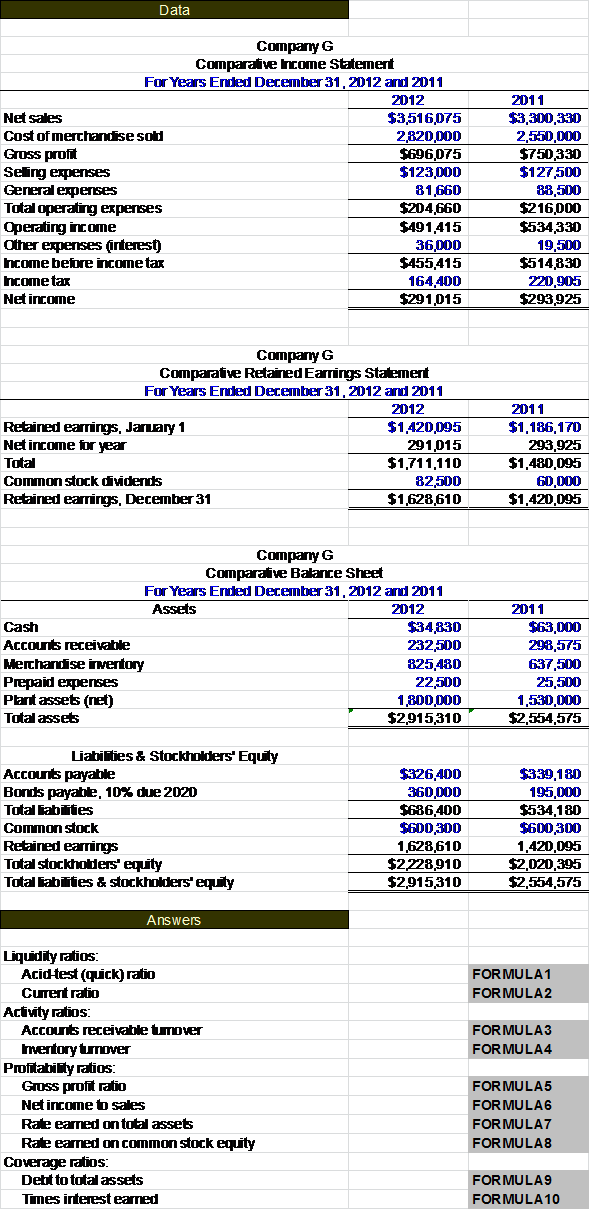Below is a brief explanation of each ratio and how to calculate it. Acid test ratio: The acid-test ratio measures how quickly a company can meet its short-term liabilities.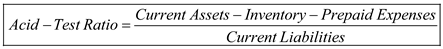Current ratio: The current ratio shows the proportion of current asset to current liabilities and indicates the company's liquidity.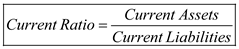Accounts receivable turnover ratio: The accounts receivable turnover ratio calculates how many times during a year a company can convert its accounts receivable into cash.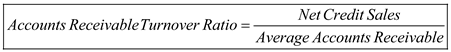Inventory turnover ratio: The inventory turnover ratio measures how many times a company's inventory is sold over a period of time.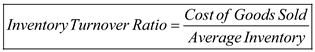Gross profit ratio: The gross profit ratio measures the percentage of gross profit to net sales.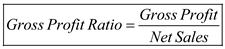Net income to sales ratio: The net income to sales ratio shows the relationship between net income and sales.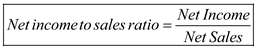Rate earned on total assets ratio: The rate earned on total assets ratio measures how well a company can have its assets produce a profit during a period of time.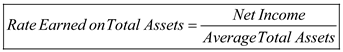Rate earned on common stock equity: Rate earned on common stock equity measures how well a company is investing the money that stockholders invest in them.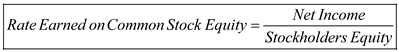Debt to total assets ratio: The debt to total assets ratio is the comparison of total debt to total assets.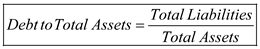Times interest earned ratio: The times interest earned ratio measures the amount of income that can be used to pay future interest expenses.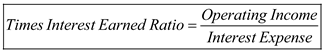Calculate the ratios with the use of following formulas: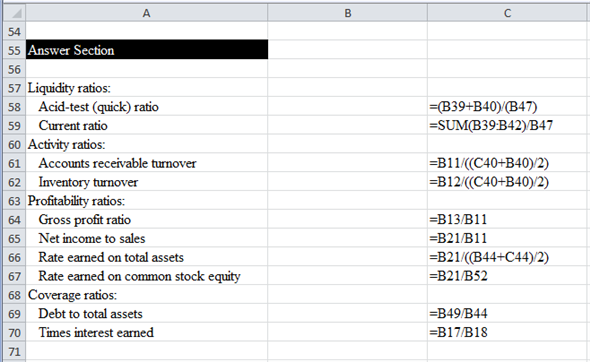Calculated ratios would be as shown below: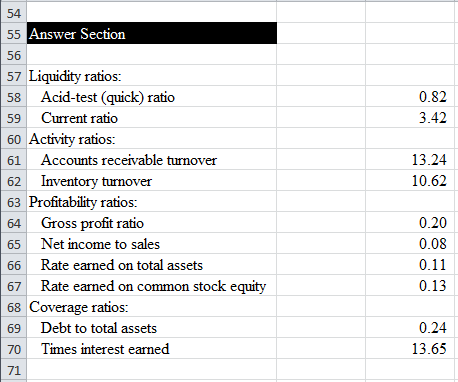Financial ratios are used for a variety of reasons and to help analyze different aspects of a company. a. The current ratio is the relationship between current assets and current liabilities. This ratio can show how liquid a company is or how easily their current assets can be converted to cash to pay its debt. b. The acid-test shows a ratio of.82. Anything under one shows that the company may not be able to pay off all its debts with only quick assets. The current ratio of 3.42 shows that it has a lot of assets that would be harder to sell off if necessary to pay debt. c. The following chart shows the comparison between Company G's financial ratios and the national average.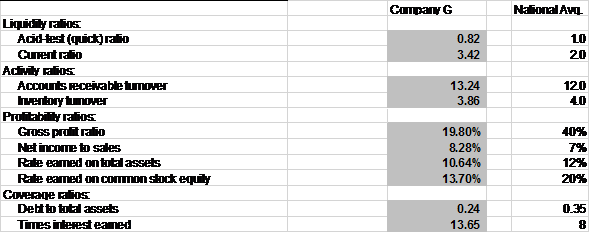In many areas Company G's numbers are very similar to the national average. The largest differences are the gross profit ratio and the rate earned on common stock equity. These numbers fall pretty significantly below the national average indicating that they are not able to sell their inventory for a high profit percentage as well as shows investors that the company may not be using their money in an efficient manner.